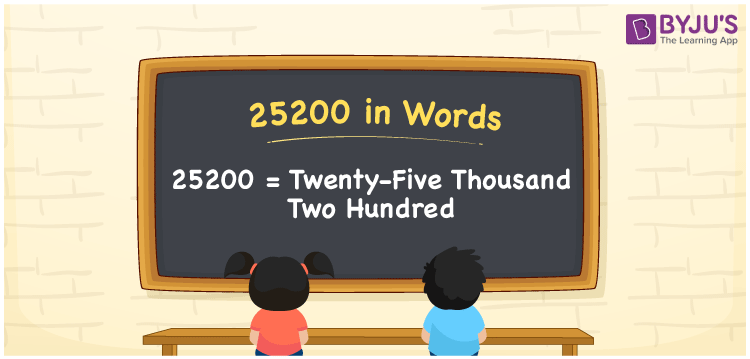# 25200 in Words

Suppose a journal contains 25200 words of content; we can say that this journal has Twenty-five thousand Two hundred words. That means we can write 25200 in words as Twenty-five thousand Two hundred. In this article, you will understand how to note the cardinal number 25200 in word form with the help of a place value chart.

 25200 in words Twenty-five thousand Two hundred Twenty-five thousand Two hundred in Numbers 25200

## 25200 in English words

Generally, we can write numbers in words using the English alphabet. Thus, we can spell 25200 in English as “Twenty-five thousand Two hundred”.## How to Write 25200 in Words?

To derive the word form of the number 25200, we need to make a place value chart with five columns since it has five digits, as shown below.

 Ten thousands Thousands Hundreds Tens Ones 2 5 2 0 0

Here, ones = 0, tens = 0, hundred = 2, thousands = 5, ten thousands = 2

By expanding these digits according to their place values, we get;

2 × Ten thousand + 5 × Thousand + 2 × Hundred + 0 × Ten + 0 × One

= 2 × 10000 + 5 × 1000 + 2 × 100 + 0 × 10 + 0 × 1

= 20000 + 5000 + 200

= Twenty thousand + Five thousand + Two hundred

= Twenty-five thousand Two hundred

Therefore, 25200 in words = Twenty-five thousand Two hundred.

### Facts About the Number 25200

As we know, 25200 is a natural number that is the successor of 25199 and the predecessor of 25201.

25200 in words – Twenty-five thousand Two hundred

Is 25200 an odd number? – No

Is 25200 an even number? – Yes

Is 25200 a perfect square number? – No

Is 25200 a perfect cube number? – No

Is 25200 a prime number? – No

Is 25200 a composite number? – Yes

## Frequently Asked Questions on 25200 in Words

Q1

### How do you say 25200 in words?

The spelling of 25200 in English words is Twenty-five thousand Two hundred and thus, we can spell 25200 in words as “Twenty-five thousand Two hundred”.
Q2

### How do you write Rs. 25200 in words on a cheque?

On a cheque, Rs. 25200 in words is written as Twenty-five thousand Two hundred rupees only.
Q3

### How do you convert 25200 in words?

We can convert the 25200 in words as: 25200 = 20000 + 5000 + 200 = 25000 + 200 = Twenty-five thousand + Two hundred = Twenty-five thousand Two hundred.